[Contents]

Visualizing A Field that is Not the Gradient of Any Potential
John Denker

## 1  Example: Arc Length

Let’s start by considering Ferris Bueller’s car problem: Suppose you borrow a car, drive it around a complicated path, and then return it to exactly where you found it. Some things will return to their original state, but some will not:

 The X-coordinate (longitude) returns to its original value. The Y-coordinate (latitude) returns to its original value. The distance traveled is nonzero. During the trip, the odometer reading advances by some nonzero amount. It does not return to its original value.

A possible path is shown in figure 1. The total length of the loop is about 5.4 units. Along the path there are hash marks every 0.2 units.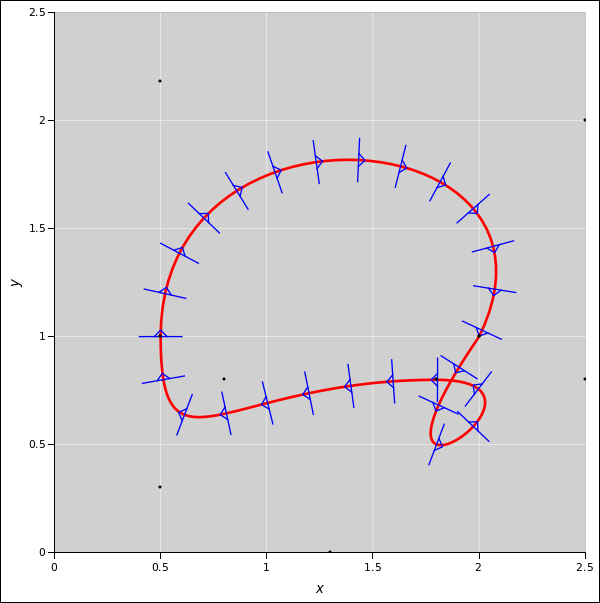Figure 1: Arc Length around a Loop

We can understand this mathematically as follows: The element of arc length (ds) is defined via:

 ds2 = dx2 + dy2
(1)

Now if we integrate around the loop, from start to finish, we find

 ∮ dx
= 0
 ∮ dy
= 0
 ∮ ds
= 5.4    (total length of path)
(2)

We can even draw a picture of dx and dy.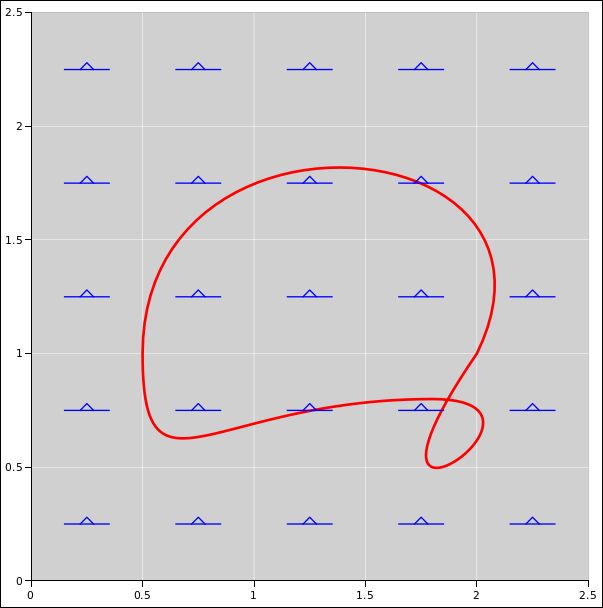Figure 2: Grady Vector Field: dx Figure 3: Grady Vector Field: dy

We see that dx and dy are very different from ds.

• We know that x is a potential, and dx is the gradient thereof. In figure 2, you can join up the hash marks to make contour lines, thereby constructing a well-behaved contour map. The height of the x-potential increases from left to right.
• Similarly, y is a potential, and dy is the gradient thereof. In figure 3, you can join up the hash marks to make contour lines, thereby constructing a well-behaved contour map. The height of the y-potential increases from bottom to top.

In contrast, s is not a potential. There is no way you can join up the hash marks in figure 1 to create a well-behaved contour map. The s-value cannot be interpreted as a height.

Forsooth, if we consider the (x,y) plane to be our state space, s is not even a function of state. Now s is a perfectly good function of s as we move along the path, but s is not a function of location in the (x,y) plane. This is particularly obvious at the point where the path crosses itself.

The same goes for ds: We know the magnitude and direction of ds as a function of s as we go along the given path, but ds not a function of location in the (x,y) plane. This is particularly obvious at the point where the path crosses itself.

Let’s be clear: s and ds exist only in the low-dimensional space defined by the path. They are not functions of state in the higher-dimensional (x,y) state space.

The moral of the story is:

• When integrating around a closed loop, do not assume the answer is zero.
• Do not assume that every vector field is the gradient of some potential.
• Do not assume that every physically-significant variable is a function of state.

## 2  Function of State, but Not the Gradient of Any Potential

An intermediate case is shown in figure 4. The hash marks represent the vector field x dy. This is intermediate in the sense that x dy is a perfectly good function of state in the (x,y) plane, so it is not as wacky as the element of arc length (ds) that we saw in figure 1. On the other hand, x dy is not the gradient of any potential, so understanding figure 4 is more work than understanding figure 2 or figure 3.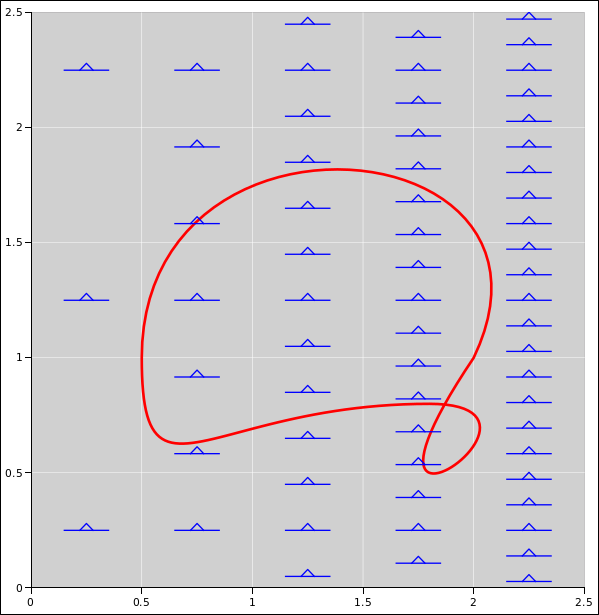Figure 4: Not the Gradient of Any Potential

In figure 4 the hash marks are partially like the contour lines on a topo map, insofar as the closeness of the hash marks indicates the steepness of the local slope. On the other hand, there is no way you can join up the hash marks to make a well-behaved contour map. At every point, there is a notion of local slope, but there is no valid notion of height. Because it is not a potential, you cannot integrate the slope to find the height in any consistent way, because the integral strongly depends on the choice of path. In particular:

 ∮ dx
= 0     (along any path)
 ∮ dy
= 0     (along any path)
 ∮ x dy
0     (along almost any path)
(including the indicated path)
(3)

This is important, because in thermodynamics it is exceedingly common to encounter vector fields of the form x dy i.e. things that are not the gradient of any potential.

Similarly, in electromagnetism, it is exceedingly common to encounter things like x dyy dx, i.e. things that are not the gradient of any potential. In particular, sometimes people who ought to know better pretend that the voltage is a potential, even when it is not. For more about the definition of voltage, including its dependence on the choice of path, see reference 1.

The moral of the story is:

• Even when it is a function of state, do not assume that every vector field is the gradient of some potential.

The diagrams in this section were created using the spreadsheet in reference 2.

## 3  Visualizing Fields That Are Not the Gradient of Any Potential

In the real world, there are lots of things that locally have a notion of “uphill” and “downhill”, but don’t have a definite height. Examples abound in electromagnetism and in thermodynamics. Figure 5 may help you visualize what’s going on. In the figure, clockwise = locally downward, everywhere.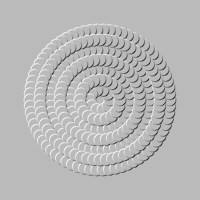Figure 5: Force Field, Not The Gradient Of Any Potential

Non-experts tend to think that every force-field that is a function of position must be derived from some potential, but it’s just not true.

If you want a real-world example, imagine you are standing near a whirlwind. If you walk clockwise around the core of the whirlwind, the force of the wind is assisting you the whole way. If you walk counterclockwise, the force of the wind is opposing you the whole way. (Walk slowly, so that any dependence on your velocity is negligible.)

In the simplest case, a whirlwind can be described as a vortex, which has a particularly simple distribution of velocities, as shown in figure 6.

• Near the core of a vortex, the velocity is high. This is indicated in the figure by having a large number of steps per unit length (for a path that goes around the center).
• Farther from the core, the velocity is lower. This is indicated by fewer steps per unit length (again, for a path that goes around the center).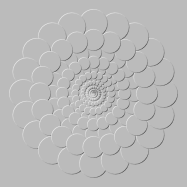Figure 6: The Velocity Field of a Vortex

It is amusing to note that in an ideal vortex, if you care only about the velocity, the integral of velocity is the same along any simply-connected path that encompasses the core, such as the red path in figure 7. In particular, the integral is 20 steps, as you can verify by counting it yourself. Meanwhile, the integral is zero for any simply-connected path that does not encompass the center, such as the blue path in figure 7.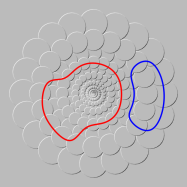Figure 7: Vortex Velocity Field, with Paths

As another example, when talking about voltage, the recommended term is simply voltage. Beware that some people use the term “electric potential” as if it were synonymous with (or preferable to) the term “voltage”, but this is a horrible misnomer and misconception. There are innumerable cases where you have a voltage but you don’t have a potential. The notorious “ground loops” and other wiring loops are a case in point. If the voltage were a potential, you wouldn’t care whether your wiring had loops or not.

While ground loops are unhelpful, many helpful devices exploit the same physics, i.e. the fact that you can pick up a voltage by going around a closed loop. Examples include transformers, generators, and betatrons. As described in reference 3, a betatron uses a magnetic field that steadily increases as a function of time. For simplicity let’s consider a simplified betatron in which the magnetic field is spatially uniform, in which case the induced electric field is shown in figure 8.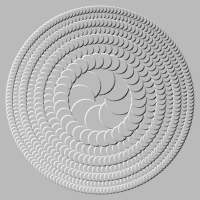Figure 8: The Electric Field in a Simplified Betatron

In figure 8 you can see that the magnitude as well as the direction of the force is changing from place to place. In contrast, in figure 5 the magnitude is the same everywhere and only the direction is changing.

The electric field inside an ordinary transformer is definitely not the gradient of any potential. It is qualitatively similar to the betatron field.

(By the way: An unsuitable example would be the frictional force due to sliding on a stationary table-top. This force is not derived from any potential. It is a function of velocity, not a function of position. We aren’t discussing such forces here.)

In figure 5 or figure 8, pick two points A and B, and a definite path from one to the other. The height-change along the path is the number of upward steps you take going along the path, minus the number of downward steps. The answer depends on your choice of path, not just on the choice of endpoints. (For a potential, such as figure 10, the answer depends only on the endpoints, independent of path.)

If we want to get fancy, we can say each of these figures represents something called a one-form, as discussed in reference 4 and references therein. A one-form that is the gradient of some potential is called grady. A one-form that is not grady is called ungrady or non-grady.

Additional terminology: A grady force field is commonly called a conservative force field. Alas, this terminology is just begging to be misunderstood. In electrodynamics, for example, both grady and non-grady force fields uphold conservation of energy, conservation of momentum, conservation of charge, et cetera, so it seems very strange to call the non-grady fields “non-conservative”.

Also: Many books refer to a grady one-form as an exact differential, and an ungrady one-form as an inexact differential. Alas, this terminology is also begging to be misunderstood. Beware that in this context, inexact does not mean imprecise or approximate. Not at all. Also beware that an inexact differential is really not a differential at all.

To summarize:

(4)

Compared to the concept of “inexact differential”, the concept of ungrady one-form is more modern, more powerful, and easier to visualize.

To be explicit: The force at each point in the field depicted by figure 5 has components given by:

 Fx = y / (x2+y2)½ Fy = −x / (x2+y2)½ Fz = 0
(5)

In this case the magnitude of F is constant everywhere, and F points clockwise everywhere.

The corresponding equations for the non-conservative force acting on the electron in the betatron are actually simpler, even though figure 8 appears more complicated:

 Fx = y Fy = −x Fz = 0
(6)

In this case, the magnitude of F is proportional to the radius. Again, F points clockwise everywhere. This field has the interesting property that it has the came amount of curl everywhere. That is, if you go clockwise around a closed loop anywhere in the betatron electric field (figure 8), the net number of downward steps that you take is proportional to the area of the loop, independent of the shape of the loop. Try it.

You can easily verify that neither of these force-fields can be the gradient of any potential. (Hint: dd Φ = 0 for any potential Φ. If you insist on writing this hint in terms of cross products, which I don’t recommend, the expression is ∇ × ∇ Φ = 0.)

A more aesthetic non-potential is presented in figure 9 but it isn’t quite as precise or generalizable.Figure 9: Waterfall, by M. C. Escher (1961)

## 4  The Fish-Scale Representation

Let’s discuss the technique used to represent one-forms in figures such as figure 8. I call this the fish-scale representation.

These fish-scale diagrams are a big help, because they are a convenient way to portray ungrady one-forms.

When talking about the forces and about the energy, we must be careful to keep track of which is which.

• A stationary electron sitting in a betatron feels a force that is a function of position. This force is perfectly well behaved. It is not the gradient of any potential.
• In contrast, the energy of the electron is not a function of position. Returning to the original position does not generally return the electron to the original energy. If you drag the electron along some path from point A to point B, the energy change is perfectly well defined, but it depends on the path. (If this were a potential, the energy change would depend only on the endpoints, independent of path.)

I don’t consider this technique an optical illusion. I consider it a non-deceptive way of portraying one-forms such as the electric field in a betatron.

The portrayal has various minor imperfections, including:

• The fish-scales portray steps whereas the real field is smooth. The steps are an unwanted artifact, a crutch that I need in order to portray what’s going on. If somebody has a way of portraying an ungrady one-form without steps, please let me know! In the meantime, you can imagine making such artifacts go away by passing to the continuum limit, as follows: Imagine a sequence of diagrams like this, where the steps become more numerous, with progressively smaller risers and progressively smaller treads between them.
• A related minor point is that the fields being represented in figure 5 and figure 8 are strictly independent of the radial coordinate, whereas the diagrams appear to have concentric rings, each alternately higher or lower than its neighbors. Equivalently, one can say there is a series of steps (up, down, up, down) along a path in the radial direction. This is an unwanted artifact, but it is minor because if you go a long distance in the radial direction, there is no cumulative up/down motion. This artifact goes away entirely in the continuum limit.

Also we must be careful when using the word "downward" (as in "clockwise = locally downward everywhere").

• There is a locally valid notion of "downward step" and "upward step”. When you cross the boundary of a scale, moving to a neighboring scale, you definitely move upward or downward.
• In contrast, the figure is not intended to portray any notion of absolute height. Any suggestion of absolute height is expressly disclaimed. If you try to label the individual disks as to absolute height, you will find that no consistent labeling is possible.

The boundary of a scale is meaningful. The question of whether one scale is above another scale is not meaningful.

The fish-scale representation works fine for grady as well as ungrady one-forms. Figure 10 shows something that is a potential, namely a conical bump. The bump has a slope, which is a grady one-form.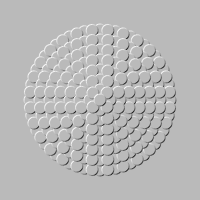Figure 10: A Force Field Derived From A Potential

Other representations are sometimes possible. Much depends on whether the state has a metric (i.e. a dot product). Consider the contrast:

 Metric No Metric

 In many situations, do have a metric. Ordinary position-space is a good example. Rectangular (Cartesian) coordinates can be used to induce a nice, simple, familiar metric. You can also use polar coordinates (in 2D or 3D), but the induced metric has singularities and other complications, and must be used with care. Also, in special relativity, spacetime has a metric, although it is slightly peculiar. And so on. On the other hand, there some situations where we lack a metric. Thermodynamics is an exceedingly important example; see reference 5. There are also situations, such as general relativity, where the metric might be unknown, or so complicated that we don’t want to deal with it.

 In such situations: The metric defines for us a notion of length and a notion of angles. We can use that to create a dual representation where every one-form corresponds to a vector and vice versa. A vector field can be represented by placing, at selected points, an arrow representing the magnitude and direction of the vector at that point. Usually the length of the arrow is used to encode the magnitude of the vector, but sometimes barbs are used instead, as discussed in reference 6. In such situations: Without a metric, there is no way to associate a one-form with a “corresponding” vector, nor vice versa. One-forms are not readily representable in terms of arrows. This makes the fish-scale representation particularly valuable.

## 5  Comparison to Other Techniques

The fish-scale technique can be considered a modification of conventional mapmakers’ techniques for depicting relief, including contour lines, shading, et cetera.

On a map drawn in two dimensions, the contours are contour lines. In three dimensions the contours are surfaces, also called shells. More generally, in a space of dimension D, the contours are hypersurfaces of dimension D−1. We note in passing that the fish-scale technique can be generalized to higher dimensions. In the D=2 fish-scale diagrams, you see lots of 3/4-complete circles. In D=3, the corresponding elements will be 3/4-complete spheres, each nestled against its neighbors. The techniques of shading to portray the orientation of the contour also generalizes. For now, though, we will concentrate on the two-dimensional case.

Plain old contour lines are problematic even for grady one-forms. For one thing, they don’t “grab” the viewer. A related problem is that contour lines don’t distinguish an upslope from a downslope. You can’t tell a pit from a peak. When cartographers want to create a map with visually perceptible relief, they must supplement (or replace) contour lines with various shading and/or color-coding schemes.

For ungrady one-forms, contours are even more problematic.

People often speak of contours as equipotentials, although technically you can’t have equipotentials if you don’t have a potential. Still, you can have the next best thing, namely constant-energy contours. Start at an arbitrary point. Shoot out “trial move” vectors in every direction. Make a note of the directions in which the trial move causes zero change in energy. Move a small distance in that direction, and iterate. This maps out the constant-energy contour. As long as you stay on this contour, your energy doesn’t change. (One thing you cannot do is label this contour as to energy, because if you leave the contour and come back to it, you will in general have a different energy.)

These constant-energy contours do a good job of portraying the orientation of a one-form even for an ungrady one-form (i.e. a non-conservative force field). However, portraying the orientation is only half the battle. Recall that we have multiple objectives:

• The orientation of the contour lines should indicate the direction of the one-form.
• The spacing between contour lines should indicate the magnitude of the one-form.

With grady one-forms, both objectives can be achieved with high accuracy. You choose contour lines that are evenly spaced in energy.

With ungrady one-forms, there is a terrible dilemma. Suppose you map out the constant-energy contours using the method described above. When you attempt to represent the magnitude of the one-form, you will find that if the spacing between contours is correct in one region it will be incorrect in neighboring regions. You will have to start new contours here and there. There is no way to do it perfectly.

Just to rub salt in the wound, the shading techniques that work for a grady one-form look hideous when applied to the discontinuous contour lines that are characteristic of an ungrady one-form.

## 6  References

1.
John Denker,
“Basic Notions of Voltage”
www.av8n.com/physics/voltage-intro.htm

2.
John Denker,
./deriv-arc-length-bezier.xls

3.
High School Teachers at CERN, “The Betatron”
https://teachers.web.cern.ch/teachers/archiv/HST2001/accelerators/teachers%20notes/betatron.htm

4.
John Denker,
“Basic Properties of Differential Forms”
www.av8n.com/physics/differential-forms.htm

5.
John Denker,
“Thermodynamics and Differential Forms”
www.av8n.com/physics/thermo-forms.htm

6.
UIUC Weather World,
“Observed Winds — represented by wind barbs”
http://ww2010.atmos.uiuc.edu/%28Gh%29/guides/maps/sfcobs/wnd.rxml
[Contents]